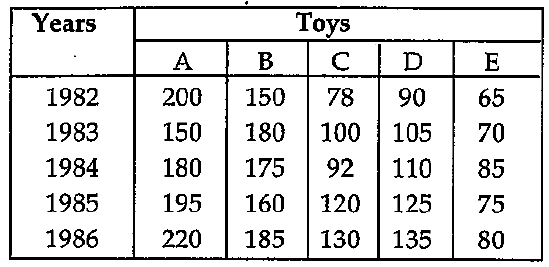# Data Interpretation - Tabular Data

>>>>>>>>Tabular Data

Instructions:

Study the following table carefully and answer the questions given below it:• A

10• B

20• C

19• D

25• Option : C
• Explanation : Production of D type toys in 1983 = 1,05,000

Production of D type toys in 1985 = 1,25,000

Percentage increase = (20000/105000) * 100

= 19.05 = 19% (app.)

Instructions:

Study the following table carefully and answer the questions given below it:

• A

C• B

B• C

A• D

D• Option : C
• Explanation : Total production of A types toys from 1982-86 = 945 thousands

Total production of B types toys from 1982-86 = 850 thousands

Total production of C types toys from 1982-86 = 520 thousands

Total production of D types toys from 1982-86 = 565 thousands

Total production of E types toys from 1982-86 = 375 thousands

Hence it was maximum for A type toys.

Instructions:

Study the following table carefully and answer the questions given below it:

• A

30• B

10• C

20• D

50• Option : B
• Explanation : Drop in production A type toys from 1982 to 1984 = 20000

Percentage drop = (20000/200000) * 100 = 10%

Instructions:

Study the following table carefully and answer the questions given below it:

• A

B• B

A• C

C• D

D• Option : D
• Explanation : From the table it is obvious that there is a continuous increaes in production of D type of toys

Instructions:

Study the following table carefully and answer the questions given below it:

• A

80• B

50• C

100• D

200• Option : B
• Explanation : If the required number is x, then by hypothesis 80,000 = x% of 1,60,000 or 80,000 = (x/100) * 160000

Therefore x = (80000 *100)/160000 = 50

Related Quiz.
Tabular Data